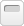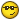• Lunes 8 de Agosto de 2022, 00:59

###Autor Tema:  MASTERMIND  (Leído 1624 veces)

#### suazo100

• Nuevo Miembro
•• Mensajes: 2##### MASTERMIND
« en: Martes 28 de Enero de 2014, 19:57 »
0
he conseguido este programa para el mastermind

MODULE CSEED
INTEGER :: SEED
INTEGER :: num
CHARACTER(LEN=1),DIMENSION(6)::gameLetters !Define allowed letters
END MODULE CSEED

PROGRAM MasterMind
USE CSEED
IMPLICIT NONE
REAL, EXTERNAL :: RANDN
INTEGER :: i,j
REAL :: x
INTEGER, DIMENSION(50) :: first = (/( 0, i=1,50)/)
CHARACTER(LEN=1), DIMENSION(4):: list
CHARACTER(LEN=1), DIMENSION(4):: gameCombination,userGuess,gamePoints
CHARACTER(LEN=4):: gamePointsChar='0000'
list =(/'G','Y','B','R'/)
gameLetters=(/'G','Y','B','R','V','S'/)
PRINT *, "Welcome to the MasterMind", "Game in Fortran"
!"*****************************************"
PRINT *, "*****************************************"
PRINT *, " Here are the rules: I choose a random "
PRINT *, " combination of 4 colors out of 6. You have "
PRINT *, " 10 tries to guess what I chose. "
PRINT *, " The colors possible are: "
PRINT *, " Green (G), Yellow (Y),Blue (, Red (R),   "
PRINT *, " Violet (V), Silver (S) "

call getGameCombination(gameCombination)
!no real need to print the game combination and discover the user
!what  it is
PRINT *,  gameCombination, " FROM OUTSIDE"
DO i=1,10
call getGuess(userGuess)
call compare(gameCombination, userGuess,gamePoints)
!Reset Scores
!gamePointsChar='0000'
DO j=1,4
gamePointsChar(j:j)=gamePoints(j)
END DO
IF (gamePointsChar=='BBBB') THEN
print *, "That's right ! ", gameCombination, " Is the guess. &
&You win after",  i, "rounds !"
exit
END IF
END DO
IF (i==10) THEN
PRINT *, "You have reached the maximu allowed tries, you lose ...&
& but you can always try again!"
END IF
END PROGRAM MasterMind

SUBROUTINE compare(gameCombination, userGuess,roundPoints)
!SUBROUTINE compare(userGuess)
use CSEED
IMPLICIT NONE
INTENT(IN) :: gameCombination, userGuess
INTENT (OUT) :: roundPoints
CHARACTER(LEN=1),DIMENSION(4):: roundPoints, gameCombination, userGuess
INTEGER :: i,j
roundPoints = (/'0','0','0','0'/)
!Just for practicing character operations roundPoints is also converted
!to a string
DO i=1,4
DO j=1,4
IF (gameCombination(i) == userGuess(i)) THEN
roundPoints(i)='B'
exit
!PRINT *, roundPoints
END IF
!else if (gameCombination(i) == userGuess(j)) THEN
if (gameCombination(i) == userGuess(j)) THEN
roundPoints(j)='W'
!PRINT *, roundPoints
!exit
END IF

!ELSE
!IF (gameCombination(i) == userGuess(j)) THEN
!    roundPoints(j)='W'
!END IF
END DO
END DO
PRINT *, "Your score " , roundPoints
!no need to give any output variable, just print scores to screen
END SUBROUTINE compare

SUBROUTINE getGameCombination(gameCombination)
! A subroutine to choose  the game combiantion from the game
! letters
USE CSEED
IMPLICIT NONE
CHARACTER(LEN=1), DIMENSION(4), INTENT (OUT):: gameCombination
REAL, EXTERNAL :: RANDN
INTEGER :: I
REAL :: Y
INTEGER, DIMENSION (8) :: T
CALL DATE_AND_TIME(VALUES = T)
SEED = T(1)+70*(T(2)+12*(T(3)+31*(T(5)+23*(T(6)+59*T(7)))))
IF (MOD(SEED,2).EQ.0) SEED = SEED-1
DO I = 1, 4
Y = RANDN()
!get numbers from 1 to 6
num = int(Y*6+1)
gameCombination(I)=gameLetters(num)
END DO
END SUBROUTINE getGameCombination

SUBROUTINE getGuess(userGuess)
!get user guess
IMPLICIT NONE
INTENT(INOUT) :: userGuess
!The subrutine has to know the shape and type of
!userGuess
CHARACTER(LEN=1), DIMENSION(4):: userGuess
!subrutine to get players guess
PRINT *, "Type your guess as 4 letters separated by spaces:"
PRINT *, 'Valid input is:  "G B S V"'
!PRINT *,'asd',userGuess
PRINT *, "Here is your guess:   ", userGuess
END SUBROUTINE getGuess

REAL FUNCTION RANDN()
! Function to generate a uniform random number in [0,1]
! following x(i+1)=a*x(i) mod c with a=7** 5 and
! c=2** 31-1.  Here the seed is a global variable.
!
USE CSEED
IMPLICIT NONE
INTEGER :: H, L, T, A, C, Q, R
DATA A/16807/, C/2147483647/, Q/127773/, R/2836/
!The DATA statement is used to specify initial values
!for variables and array elements.
H = SEED/Q
L = MOD(SEED, Q)
T = A*L - R*H
IF (T .GT. 0) THEN
SEED = T
ELSE
SEED = C + T
END IF
RANDN = SEED/FLOAT(C)
END FUNCTION RANDN

********************************************************************
pero no entiendo la funcion randn() y queria saber si existe otra forma de conseguir el numero aleatorio.He visto la subrutina RANDOM_NUMBER pero no se muy bien como utilizarla dentro de este programa